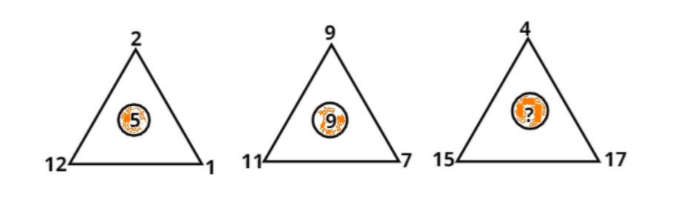Courses
Courses for Kids
Free study material
Free LIVE classes
MoreLIVE
Join Vedantu’s FREE Mastercalss

# Which number replaces the question mark?$a.{\text{ 10}} \\ b.{\text{ 12}} \\ c.{\text{ 14}} \\ d.{\text{ 16}} \\$Verified
363.9k+ views
Hint: - Addition of the corner numbers and divided by three is equal to the middle number.
As we see that the first figure follows the pattern which is addition of the corner numbers and divided by three is equal to the middle number.

$\therefore 5 = \dfrac{{2 + 12 + 1}}{3} \\ \therefore 5 = \dfrac{{15}}{3} \\ \therefore 5 = 5 \\$
Which is true.
Second figure also follows the pattern which is addition of the corner numbers and divided by three is equal to the middle number.
$\therefore 9 = \dfrac{{9 + 11 + 7}}{3} \\ \therefore 9 = \dfrac{{27}}{3} \\ \therefore 9 = 9 \\$
Which is true.
Therefore third figure also follow the same pattern
$\therefore ? = \dfrac{{4 + 15 + 17}}{3} = \dfrac{{36}}{3} = 12$
So, 12 replaces the question mark.
Hence, option (b) is correct.

Note: - Whenever we face such types of questions first determine which pattern is followed by the two figures, so the third figure also follows the same pattern, and then we will easily calculate which number is replaced by question mark.

Last updated date: 21st Sep 2023
Total views: 363.9k
Views today: 5.63k